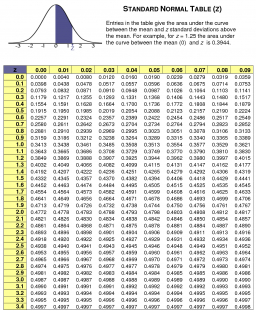# Z-score

The mean adult male pulse rate is 67.3 beats per minute with a standard deviation of 10.3. Find the z-score for an adult male’s pulse rate of 75. (Round the z-score to two decimal places. )Did you find an error or inaccuracy? Feel free to write us. Thank you!Tips to related online calculators
Looking for help with calculating arithmetic mean?
Looking for a statistical calculator?
Looking for a standard deviation calculator?

#### You need to know the following knowledge to solve this word math problem:

We encourage you to watch this tutorial video on this math problem: